# 第二章－技术面分析－(一)蜡烛图(Ｋ线图)交易方法-下

2013年03月12日

5. 镊型顶形态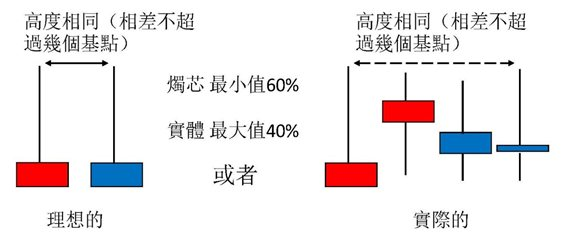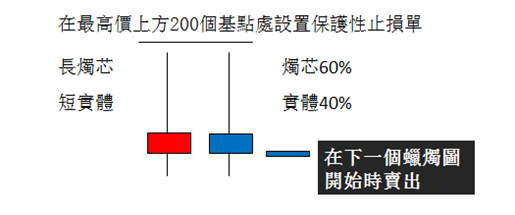a.取得自己所需要的线图，2012417日的价格K线呈现形顶的形态。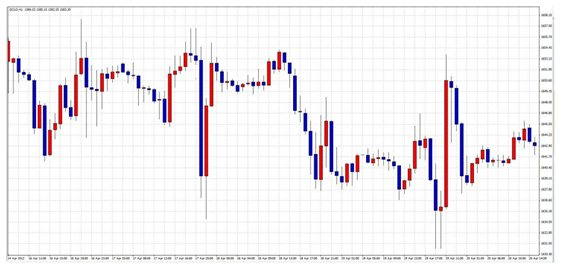b.入市位置 = 1653、止损位置 = 1657止赢位置 = 1641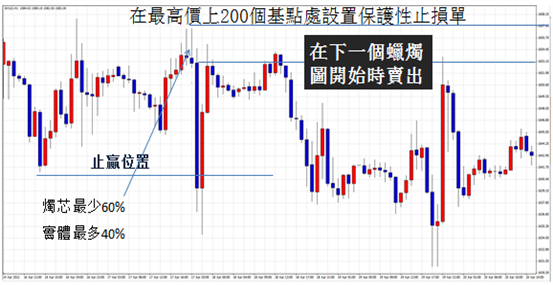c.空单入市风险评估：由于是做空，当价格下跌时，获利增加，因为无法预期跌是延续多久，以前一波支撑点为止赢点，按造公式计算，数值为负数，然而，意思和做多的意思相同，亦即做空的负数可视为正数比率。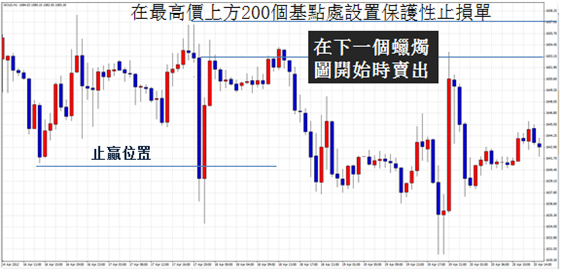＊风险 =入市位置 -损位置  = 1653.15 -1657.15 = -4

＊获利 =止赢位置 -入市位置  = 1641-1653.15 = -12.15

＊风险比率 -4 : -12.15= 1 : 4.075

6.型底形态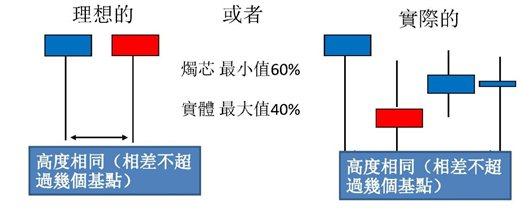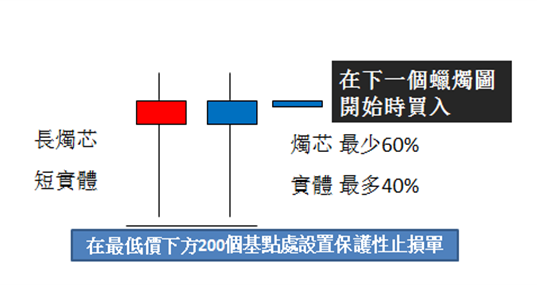a.取得自己所需要的线图，2012118日的价格K线呈现形底的形态。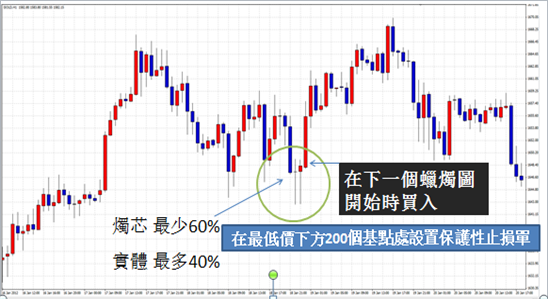1. 入市位置 = 1648.00、止损位置 = 1642.00止赢位置 = 1667.00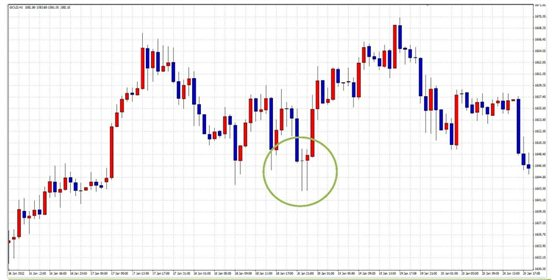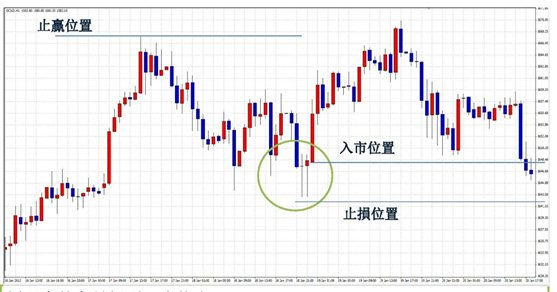c.入市风险评估

＊风险 =入市位置 -损位置  = 1648 -1642 = 5

＊获利 =止赢位置 -入市位置  = 1667-1642 = 25

＊风险比率 5 : 25  = 1 : 5

7.多头吞噬蜡烛图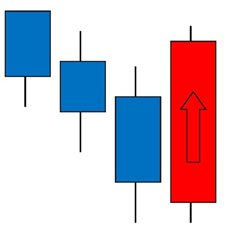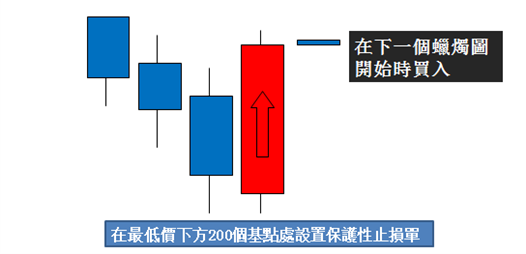a.取得自己所需要的线图，2011527日的价格K线图呈现多头吞噬的形态。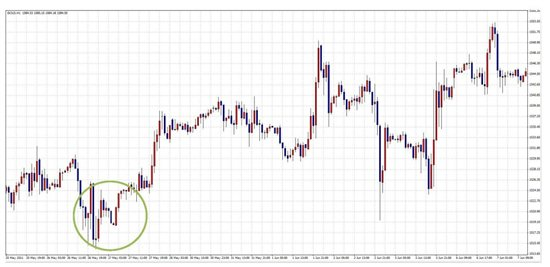b.入市位置 = 1521.50、止损位置 = 1518.50止赢位置 = 1530.50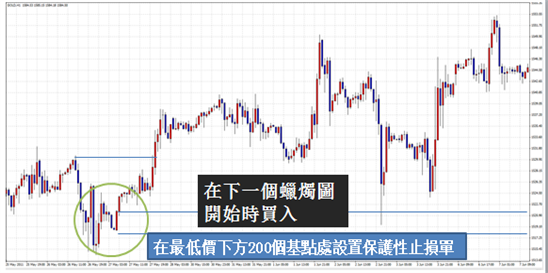c.入市风险评估

8.空头吞噬蜡烛图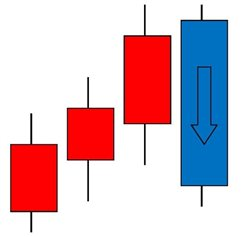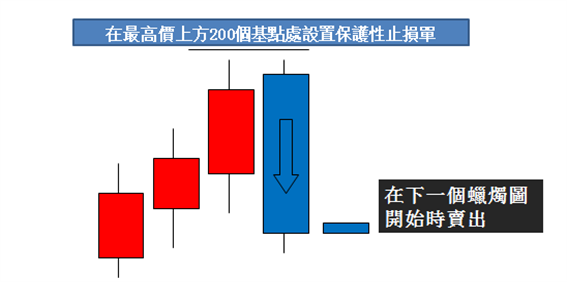a.取得自己所需要的线图，2012522日的价格K线图呈现空头吞噬的形态，如图。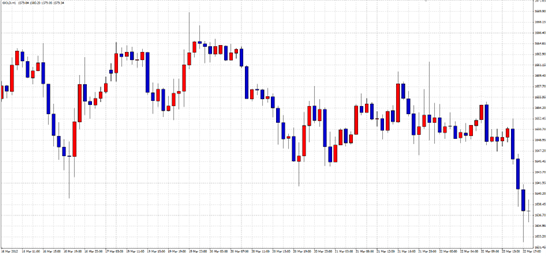b.先找出：入市位置、止损位置、止赢位置；在高价上方50个基点处设置保护性指损单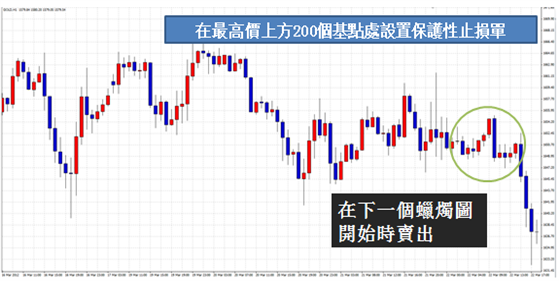c.入市位置 = 1649、止损位置 = 1655止赢位置 = 1633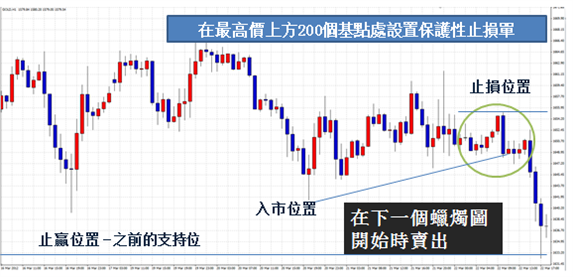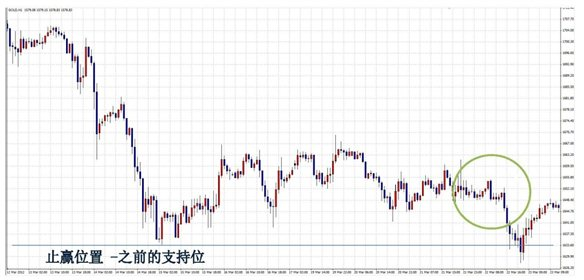d.入市风险评估

＊风险 =损位置 -入市位置  = 1655 -1649 = 6

＊获利 =入市位置 -止赢位置  = 1655 -1633 = 22

＊风险比率 6 : 22   = 1 : 3.6